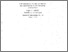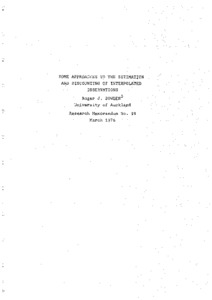# some approaches to the estimation and discounting of interpolated observations

Bowden, Roger J. (March 1976) some approaches to the estimation and discounting of interpolated observations. Closed Series > Forschungsberichte / Research Memoranda 98Preview
Text
fo98.pdf

Download (1MB) | Preview

## Abstract or Table of Contents

abstract: the interpolation of economic time series has a long and consistently unfashionable history as an object of study. nevertheless economists have from time to time been forced by the inadequacy of their data or the ambition of their constructs to consider ways of filling in the gaps where observations are missing. the problem known as "missing observations" has been fairly intensively researched in the statistical literature (for a review, see a.a. afifi and e.m. elashoff (1966)). the tenor of this work has been to design parameter estimators which compensate, as it were, for the missing data, rather than stressing the estimation of the missing observations themselves. the economist, however, is often interested in testing a numberof alternative hypotheses, perhaps of differing functional form. to design a different estimation procedure for each particular hypothesis under test would represent a substantial and possibly unjustified use of research time. thus it is useful to be able to estimate in a relatively simple way the missing observations themselves, and once having achieved the complete series, to know what modifications must be made to standard tests in screening a number of alternative hypotheses. there are thustwo related tasks to which the present paper addresses itself. the first is to find a relatively simple and general method of estimation of the observations to be interpolated. the use of a related series for which observations are readily availableon the required basis was investigated by m. friedman (1962). g.c. chow and an-loh lin (1971) showed how to formalize and generalize this work in the context of least-squares estimators. to complement this work we need now to pay some attention to methods based on likelihood principles. the method of maximum-likelihood was applied by m. drettakis (1973) in a missing-observations study, to generate estimates of observations missing at the start of a series for a simultaneous equation model. in the present paper we study the more usual situation where observations are missing in a regular way throughout the series. although we investigate briefly a method based on true maximum likelihood principles, we reject at in favour of a "quasi-likelihood" procedure which perhaps has more in common with bayesian techniques. given that one has obtained point estimates with associated variances or covariances, there remains the problem of how the now-complete data series is to be deployed. under the sort of research strategy described above, we might be interested in running regressions in order to test a set of different hypotheses. intuition suggests that tests based on interpolated data ought not to be quite so conclusive as those employing a complete series. in other words, interpolated data should be discounted in some way, a problem which has not, to this author's knowledge, been raised in a systematic manner. as we shall see, the problem of estimation, on the one hand, and discounting, on the other, are intimately related. for the starting point for discounting formulas are the variances and covariances of the interpolations as yielded by the method of estimation. more than this, the approach adopted to the problem of estimation is of importance for the specification of the regression models, and hence for the meaning of the discounting process. we consider in this paper the problem of interpolation, strictly defined. typically this arises in connection with a stock series, such as the quantity of money at a particular point in time. the particular example we employ is the problem of interpolating observations on the net worth (wealth) of a community. a related problem (see chow and lin (1971)) is that of distribution, typically applicable to a flow series. thus an available quarterly income series may have to be assigned to monthly observations, under the constraint that the sum of the monthly observations should add to the given monthly total. the limitation we impose in discussing only the pure interpolation problem is necessitated by space requirements, although we think that similar techniques, appropriately modified for the adding-up constraint, could be applied to the distribution problem. the scheme of the paper is as follows. in section i we interpret and compare two distinct approaches based on the likelihood function. we work in terms of a linear autoregressive process of the first order in which every second observation is to be interpolated. to infuse our discussion with some living substance we cast it as the problem of interpolating annual wealth observations. in section ii we extend this basic work to cover (a) higher-order interpolation - say, every second and third observation to be interpolated (b) higher-order autoregressive formulas and (c) to the case where interpolative formula may be non-linear, based in this instance on a more realistic approach to the problem of interpolating a wealth series. we include also (d) a short discussion of some sampling problems. finally, section iii contains the ideas and techniques associated with the problem of discounting the interpolated data in carrying out regressions.;

Item Type: IHS Series Published 26 Sep 2014 10:34 01 Apr 2016 14:07 https://irihs.ihs.ac.at/id/eprint/98

### Actions (login required)View Item